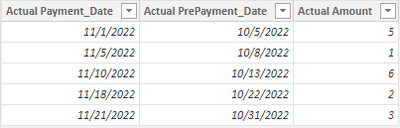cancel
Showing results for
Did you mean:

Fabric is Generally Available. Browse Fabric Presentations. Work towards your Fabric certification with the Cloud Skills Challenge.Impactful Individual

## Cumulative signal with userelationship

Hi,

I have 2 datasets:
1. Calendar

2. Table with cash and dates (with different type of dates like, prepayment date, payment, etc)

So, i can't do a relationhsip with the calendar, becasue the relation will be for many columns in the table of cash

To solve that sum of values for each period or type of date, i have created the following measures and they work fine.

``````Actual Amount using PrePayment Date = CALCULATE(Cash[Actual Amount], USERELATIONSHIP(Cash(CalendarCash[Date],Cash[Actual PrePayment_Date]))

Actual Amount using Payment Date = CALCULATE(Cash[Actual Amount], USERELATIONSHIP(Cash(CalendarCash[Date],Cash[Actual Payment_Date]))``````

So i have a tablix matrix with calendar dates, and then use one or the other and they work with the same calendar.

The issue is when i try to do accumulated values from that measure that it seems not work
i have tried and it doesnt work.

``````SUMX (
FILTER (
ALLSELECTED ( Cash[Actual PrePayment_Date] ),
CDB_CashMovements[Actual PrePayment_Date] <= MAX ( CDB_CashMovements[Actual PrePayment_Date] )
),
CDB_CashMovements[Actual Amount using PrePayment Date]
)``````

or

``````CALCULATE (
Cash[Actual Amount using PrePayment Date],
FILTER (
ALLSELECTED ( cash[Actual PrePayment_Date],
cash[Actual PrePayment_Date] <= MAX ( cash[Actual PrePayment_Date] )
)
)``````

but they dont do the accumulated value of cash.

Can someone help me on this?

Regards!

Did I answer your question? Mark my post as a solution! Appreciate with a Kudos!! (Click the Thumbs Up Button)
1 ACCEPTED SOLUTIONCommunity Support

Hi @dobregon ,

According to your description, I create a sample.

Cash table:CalendarCash table:The two tables have two inactive relationship as you designed. Here's my solution.

1.I create two measure similar to yours.

``````Actual Amount using Payment Date =
CALCULATE (
SUM ( Cash[Actual Amount] ),
USERELATIONSHIP ( Cash[Actual Payment_Date], CalendarCash[Date] )
)
``````
``````Actual Amount using PrePayment Date =
CALCULATE (
SUM ( Cash[Actual Amount] ),
USERELATIONSHIP ( Cash[Actual PrePayment_Date], CalendarCash[Date] )
)
``````

2. I create two accumulate measures.

``````Accumulate Payment =
IF (
[Actual Amount using Payment Date] = BLANK (),
BLANK (),
SUMX (
FILTER (
ALLSELECTED ( 'CalendarCash'[Date] ),
'CalendarCash'[Date] <= MAX ( 'CalendarCash'[Date] )
),
[Actual Amount using Payment Date]
)
)
``````
``````Accumulate PrePayment =
IF (
[Actual Amount using PrePayment Date] = BLANK (),
BLANK (),
SUMX (
FILTER (
ALLSELECTED ( 'CalendarCash'[Date] ),
'CalendarCash'[Date] <= MAX ( 'CalendarCash'[Date] )
),
[Actual Amount using PrePayment Date]
)
)
``````

If you want to show all days of the calendar date, you can remove IF statement in the formula, only keep else part. Put Date from CalendarCash table and other measures in a matrix, get the correct result:I attach my sample below for your reference.

Best Regards,
Community Support Team _ kalyj

If this post helps, then please consider Accept it as the solution to help the other members find it more quickly.

2 REPLIES 2Community Support

Hi @dobregon ,

According to your description, I create a sample.

Cash table:CalendarCash table:The two tables have two inactive relationship as you designed. Here's my solution.

1.I create two measure similar to yours.

``````Actual Amount using Payment Date =
CALCULATE (
SUM ( Cash[Actual Amount] ),
USERELATIONSHIP ( Cash[Actual Payment_Date], CalendarCash[Date] )
)
``````
``````Actual Amount using PrePayment Date =
CALCULATE (
SUM ( Cash[Actual Amount] ),
USERELATIONSHIP ( Cash[Actual PrePayment_Date], CalendarCash[Date] )
)
``````

2. I create two accumulate measures.

``````Accumulate Payment =
IF (
[Actual Amount using Payment Date] = BLANK (),
BLANK (),
SUMX (
FILTER (
ALLSELECTED ( 'CalendarCash'[Date] ),
'CalendarCash'[Date] <= MAX ( 'CalendarCash'[Date] )
),
[Actual Amount using Payment Date]
)
)
``````
``````Accumulate PrePayment =
IF (
[Actual Amount using PrePayment Date] = BLANK (),
BLANK (),
SUMX (
FILTER (
ALLSELECTED ( 'CalendarCash'[Date] ),
'CalendarCash'[Date] <= MAX ( 'CalendarCash'[Date] )
),
[Actual Amount using PrePayment Date]
)
)
``````

If you want to show all days of the calendar date, you can remove IF statement in the formula, only keep else part. Put Date from CalendarCash table and other measures in a matrix, get the correct result:I attach my sample below for your reference.

Best Regards,
Community Support Team _ kalyj

If this post helps, then please consider Accept it as the solution to help the other members find it more quickly.Impactful Individual

@v-yanjiang-msft  thanks a lot, that works!!!!!

Did I answer your question? Mark my post as a solution! Appreciate with a Kudos!! (Click the Thumbs Up Button)Announcements#### Power BI Monthly Update - November 2023

Check out the November 2023 Power BI update to learn about new features.#### The largest Power BI and Fabric virtual conference

130+ sessions, 130+ speakers, Product managers, MVPs, and experts. All about Power BI and Fabric. Attend online or watch the recordings.Top Solution Authors
Top Kudoed Authors
Users online (6,147)